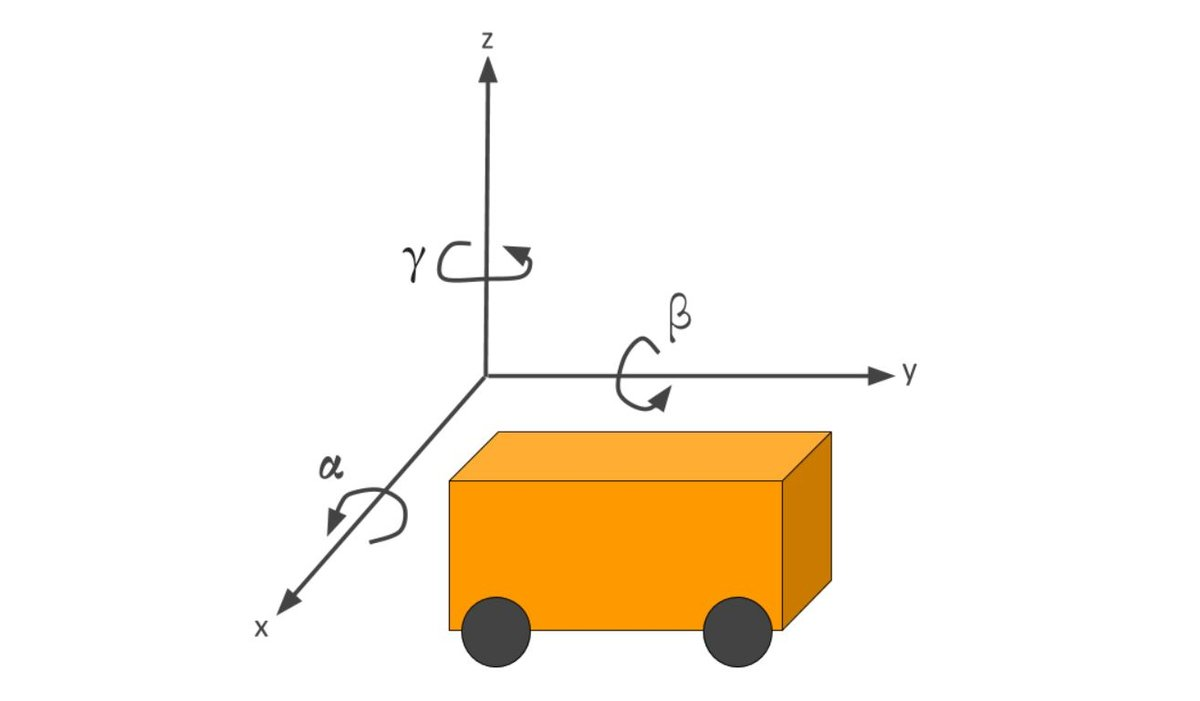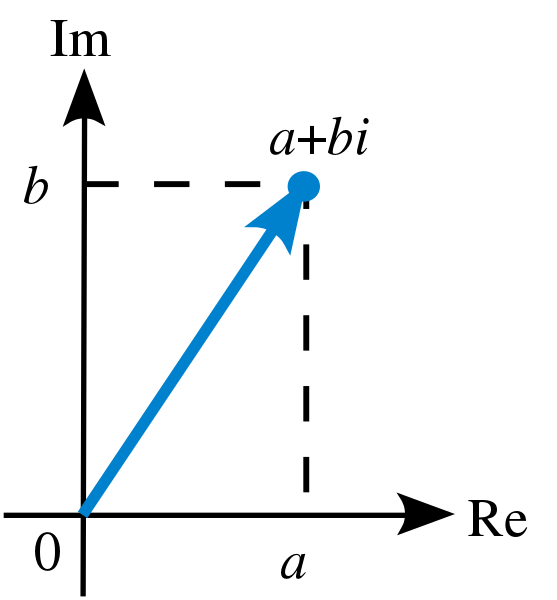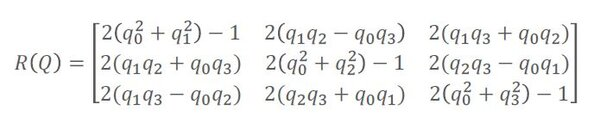# How to Convert a Quaternion to a Rotation MatrixIn this tutorial, I’ll show you how to convert a quaternion to a three-dimensional rotation matrix. At the end of this post, I have provided the Python code to perform the conversion.

# What is a Quaternion?

A quaternion is one of several mathematical ways to represent the orientation and rotation of an object in three dimensions. Another way is to use Euler angle-based rotation matrices like I did on this post and this post (i.e. roll, pitch, and yaw), as well as the cover image of this tutorial.

Quaternions are often used instead of Euler angle rotation matrices because “compared to rotation matrices they are more compact, more numerically stable, and more efficient” (Source: Wikipedia).

Note that a quaternion describes just the rotation of a coordinate frame (i.e. some object in 3D space) about an arbitrary axis, but it doesn’t tell you anything about that object’s position.

# The Use of Quaternions in Robotics

Quaternions are the default method of representing orientations and rotations in ROS, the most popular platform for robotics software development.

In robotics, we are always trying to rotate stuff. For example, we might observe an object in a camera. In order to get a robotic arm to grab the object, we need to rotate the camera reference frame to the robot reference frame so that the robot “knows” the location of the object in its own coordinate frame.

Once the rotation from camera pixel coordinates to robot base frame coordinates is complete, the robotic arm can then move its motors to the appropriate angles to pick up the object.

# How to Represent Quaternions

Quaternions are an extension of complex numbers. However instead of two values (e.g. a + bi or x + yi…same thing) that represent a point (or vector), we have four values (a, b, c, d):

q = a + bi + cj + dkVisualizing a point (a, b) as a complex number on a two-dimensional Argand diagram. Source: Wikipedia

The four values in a quaternion consist of one scalar and a 3-element unit vector.

Instead of a, b, c, and d, you will commonly see:

q = w + xi + yj + zk or q = q0 + q1i + q2j + q3k

• q0 is a scalar value that represents an angle of rotation
• q1, q2, and q3 correspond to an axis of rotation about which the angle of rotation is performed.

Other ways you can write a quaternion are as follows:

• q = (q0, q1, q2, q3)
• q = (q0, q) = q0 + q

The cool thing about quaternions is they work just like complex numbers. In two dimensions, you can rotate a vector using complex number multiplication. You can do the same with quaternions. The math is more complicated with four terms instead of two, but the principle is the same.

Let’s take a look at a two-dimensional example of complex number multiplication so that you can understand the concept of multiplying imaginary (complex) numbers to rotate a vector. Quaternions add a couple more variables to extend this concept to represent rotation in the 3D space.

# 2D Example

Suppose we have a vector on a 2D plane with the following specifications: (x = 3, y = 1).

This vector can be represented in complex numbers as:

3 + i (e.g. using the x +yi form of complex numbers)

Let’s rotate this vector 45 degrees (which is π/4 in radians).

To rotate 45 degrees, we multiply the number by:

cos(π/4) + sin(π/4)i (De Moivre’s formula)

So, we have sqrt means (“take the square root of”):

(1/sqrt(2)+ i/sqrt(2)) * (3 + i) = sqrt(2) + 2sqrt(2)i

And since:

sqrt(2) = 1.414

our new vector is:

(x = 1.414, y = 4.242)

As I mentioned earlier, the math for multiplying real quaternions together is more complex than this, but the principle is the same. Multiply an orientation (represented as a quaternion) by a rotation (represented as a quaternion) to get the new orientation.

# Convert a Quaternion to a Rotation Matrix

Given a quaternion, you can find the corresponding three dimensional rotation matrix using the following formula.Source: Quaternions and Rotation Sequences: A Primer with Applications to Orbits, Aerospace and Virtual Reality by J. B. Kuipers (Chapter 5,  Section 5.14 “Quaternions to Matrices”, pg. 125)

## Python Code

In Python code, we have:

```import numpy as np

def quaternion_rotation_matrix(Q):
"""
Covert a quaternion into a full three-dimensional rotation matrix.

Input
:param Q: A 4 element array representing the quaternion (q0,q1,q2,q3)

Output
:return: A 3x3 element matrix representing the full 3D rotation matrix.
This rotation matrix converts a point in the local reference
frame to a point in the global reference frame.
"""
# Extract the values from Q
q0 = Q
q1 = Q
q2 = Q
q3 = Q

# First row of the rotation matrix
r00 = 2 * (q0 * q0 + q1 * q1) - 1
r01 = 2 * (q1 * q2 - q0 * q3)
r02 = 2 * (q1 * q3 + q0 * q2)

# Second row of the rotation matrix
r10 = 2 * (q1 * q2 + q0 * q3)
r11 = 2 * (q0 * q0 + q2 * q2) - 1
r12 = 2 * (q2 * q3 - q0 * q1)

# Third row of the rotation matrix
r20 = 2 * (q1 * q3 - q0 * q2)
r21 = 2 * (q2 * q3 + q0 * q1)
r22 = 2 * (q0 * q0 + q3 * q3) - 1

# 3x3 rotation matrix
rot_matrix = np.array([[r00, r01, r02],
[r10, r11, r12],
[r20, r21, r22]])

return rot_matrix
```